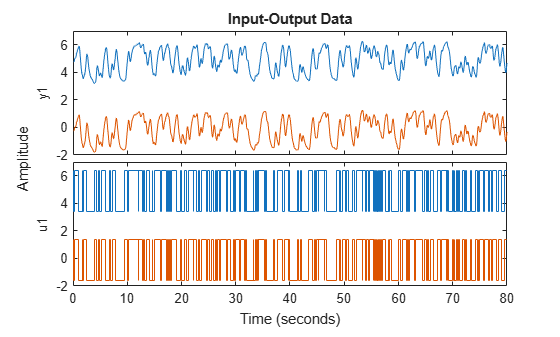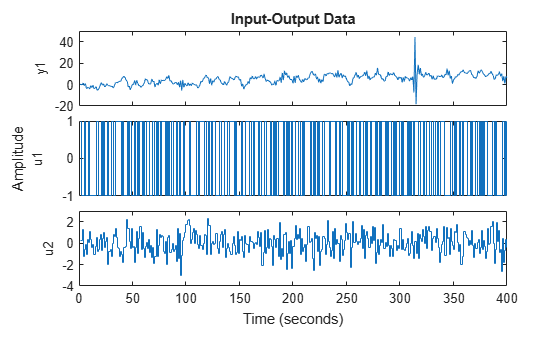# getTrend

Create trend information object to store offset, mean, and trend information for time-domain signals stored in `iddata` object

## Syntax

``T = getTrend(data)``
``T = getTrend(data,0)``
``T = getTrend(data,1)``

## Description

example

````T = getTrend(data)` constructs a `TrendInfo` object to store offset, mean, or linear trend information for detrending or retrending data. You can assign specific offset and slope values to `T`. You can then apply the trend information in `T` to either `data` or to other `iddata` objects by using `detrend` or `retrend`.```

example

````T = getTrend(data,0)` computes the means of input and output signals and stores them as the `InputOffset` and `OutputOffset` properties of `T`, respectively.```

example

````T = getTrend(data,1)` computes a best-fit straight line for both input and output signals and stores them as properties of `T`. The following code represents the straight line:ULine = Tr.InputOffset + (time-t0)*Tr.InputSlope YLine = Tr.OutputOffset + (time-t0)*Tr.OutputSlopeHere, `time` is equal to `Z.SamplingInstants` and `t0` is equal to `data.Tstart`.```

## Examples

collapse all

Remove a specified offset from input and output signals.

Load SISO data containing vectors `u2` and `y2`.

`load dryer2`

Create a data object with a sample time of 0.08 seconds and plot it.

```data = iddata(y2,u2,0.08); plot(data)```The data has a nonzero mean value.

Store the data offset and trend information in a `TrendInfo` object.

`T = getTrend(data);`

Assign offset values to the `TrendInfo` object.

```T.InputOffset = 5; T.OutputOffset = 5;```

Subtract the offsets from the data.

`data_d = detrend(data,T);`

Plot the detrended data on the same plot.

```hold on plot(data_d)```View the mean value removed from the data.

`get(T)`
```ans = struct with fields: DataName: 'data' InputOffset: 5 OutputOffset: 5 InputSlope: 0 OutputSlope: 0 ```

Compute input-output signal means, store them, and detrend the data.

Load SISO data containing vectors `u2` and `y2`.

`load dryer2`

Create a data object with a sample time of 0.08 seconds.

`data = iddata(y2,u2,0.08);`

Compute the mean of the data.

`T = getTrend(data,0);`

Remove the mean from the data.

`data_d = detrend(data,T);`

Plot the original and detrended data on the same plot.

`plot(data,data_d)`Load and plot data that contains two input channels and one output channel.

```load z7lintrend z7L plot(z7L)```The output channel of `z7L` contains a linear trend that is not present in the input channels. Compute the trend information.

`T = getTrend(z7L,1)`
```Trend specifications for data "z7L" with 2 input(s), 1 output(s), 1 experiment(s): DataName: 'z7L' InputOffset: [-0.0764 -0.0683] OutputOffset: -0.2642 InputSlope: [4.8338e-04 3.1642e-04] OutputSlope: 0.0268 ```

Limit the trend information to the output channel only by setting the input trend values to 0.

```T.InputOffset = [0 0]; T.InputSlope = [0 0]; T```
```Trend specifications for data "z7L" with 2 input(s), 1 output(s), 1 experiment(s): DataName: 'z7L' InputOffset: [0 0] OutputOffset: -0.2642 InputSlope: [0 0] OutputSlope: 0.0268 ```

Remove the linear trend from the data.

```z7d = detrend(z7L,T); plot(z7d)```The trend is no longer in the output data and the input data is unchanged.

## Input Arguments

collapse all

Time-domain input-output data, specified as an `iddata` object containing one or more sets of time-domain signals. The `iddata` object can contain SISO, MIMO, or multiexperiment data. The signal sets can contain either input and output data or output data only.

## Output Arguments

collapse all

Trend information, returned as a `TrendInfo` object.

## Version History

Introduced in R2009a